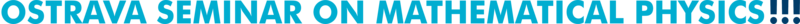Past talks (2018)
 35. February 13, 2018 František Štampach (Czech Technical University in Prague) On the asymptotic eigenvalue distribution of Toeplitz matrices and generalizations Abstract: In the first part of the talk, we give a review on the classical topic concerning the asymptotic eigenvalue distribution of a sequence of Toeplitz matrices when the size of the matrix tends to infinity. The second part will be devoted to certain generalizations of the Toeplitz matrices studied by Kac, Murdock, and Szego. We address the same problem on the eigenvalue distribution for these matrices, show some partial results and open problems. Slides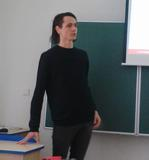36. February 27, 2018 Jan Boronski (University of Ostrava) Recent advances on minimal systems and minimal spaces Abstract: A minimal dynamical system is the one in which the trajectory of every point is dense in the space. In this case the space is called minimal. In my talk I shall discuss some of my recent results concerning minimality, including the construction of a family of embeddings of adding machines (odometers) into the real line, with vanishing derivative everywhere. Slides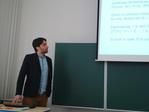37. March 6, 2018 Ondřej Turek (University of Ostrava and Nuclear Physics Institute, Czech Academy of Sciences) A generalization of circulant Hadamard and conference matrices Abstract: An Hadamard matrix is a square matrix with entries 1 and -1 such that its rows are mutually orthogonal. The so-called circulant Hadamard conjecture states that a circulant matrix of order n>1 can be Hadamard only for n=4. The conjecture, which dates back to 1963, was partially proved for symmetric circulant matrices by Johnsen in 1964; the general case, however, remains open. A conference matrix is a square matrix with entries 0 on the diagonal, 1 and -1 off the diagonal, and having mutually orthogonal rows. In 1976, Stanton and Mullin showed that a circulant conference matrix exists only of order n=2. In the talk, we introduce a common generalization of the two types of matrices by setting the diagonal entries equal to a parameter d, while keeping the orthogonality condition and the requirement that the off-diagonal entries belong to {-1,1}. We ask when such a matrix of order n>1 can be circulant. Proving that the order must be n=2d+2 whenever the matrix is symmetric or d is even, we generalize both the theorem of Johnsen (1964) and the theorem of Stanton and Mullin (1976) at the same time. Finally, we conjecture that the relation n=2d+2 is valid also when d is odd, which generalizes the circulant Hadamard conjecture. The talk is based on a joint work with D. Goyeneche. Slides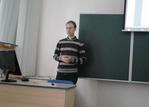38. March 15, 2018 Julius Lang (University of Jena) Finsler metrics with three dimensional projective symmetry algebra in dimension 2 Abstract: We consider the Finslerian version of a problem posed by Sophus Lie in 1882: Describe metrics admitting at least two independent projective symmetries. A projective symmetry is a vector field, whose flow maps geodesics to geodesics as unparametrized curves. The set of projective symmetries forms a Lie algebra $$p(F)$$. In dimension two, if $$dim(p)>3$$, then $$dim(p)=8$$ and $$F$$ is projectively flat. We will deal with the next interesting case, i.e. Finsler metrics $$F$$ with $$dim(p)=3$$ and prove that such a Finsler metric $$F$$ is projectively equivalent to a Randers metric $$F = \sqrt{g} + b$$ with $$p(F)=iso(g)$$, or a Riemannian metric in some local coordinates. This is done by first describing all possible systems of unparametrized geodesics with three projective symmetries and then constructing for each of them a Finsler function. If time permits, we discuss possible approaches on how to improve this result: How can one describe all Finsler functions with a predescribed system of unparametrized geodesics? We might also have a glance in the case where $$dim(p)=2$$. Slides   Handouts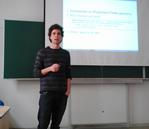39. March 28, 2018 Andrii Khrabustovskyi (Graz University of Technology) Spectral properties of periodic Schrödinger operators with $$\delta'$$-potentials Abstract: We consider a family $$\{\mathcal{H}_\varepsilon\}_{\varepsilon}$$ of $$\varepsilon\mathbb{Z}^n$$-periodic Schrödinger operators with $$\delta'$$-interactions supported on a lattice of closed compact surfaces; within a minimal period cell one has $$m\in\mathbb{N}$$ surfaces. We show that in the limit when $$\varepsilon\to 0$$ and the interactions strengths are appropriately scaled, $$\mathcal{H}_\varepsilon$$ has at most $$m$$ gaps within finite intervals, and moreover, the limiting behavior of the first $$m$$ gaps can be completely controlled through a suitable choice of those surfaces and of the interactions strengths. This is a joint work with Pavel Exner. Slides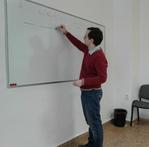40. April 6, 2018 Jiří Lipovský (University of Hradec Králové and Nuclear Physics Institute, Czech Academy of Sciences) Smilansky-Solomyak model with a $$\delta'$$-interaction Abstract: Uzy Smilansky proposed a model of irreversible dynamics, in which a quantum graph is coupled to a harmonic oscillator. We study the case of a line Laplacian coupled to a harmonic oscillator via the $$\delta'$$-interaction, i.e. the Hamiltonian formally written as $\mathbf{H}_\beta = -\frac{\partial^2}{\partial x^2} + \frac{1}{2}\left(-\frac{\partial^2}{\partial y^2}+y^2\right) + \frac{\beta}{y}\,\delta'(x)\,.$ Similarly to the previously studied case of a $$\delta$$-interaction, the spectrum behaves differently for the coupling constant $$\beta>2\sqrt{2}$$, $$\beta=2\sqrt{2}$$, and $$\beta<2\sqrt{2}$$. We investigate both the absolutely continuous spectrum and the discrete spectrum and its asymptotical properties. This is a joint work with Prof. P. Exner, based on the paper: P. Exner, J. Lipovský, Smilansky-Solomyak model with a $$\delta'$$-interaction, arXiv:1801.08304. Slides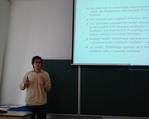41. May 22, 2018 Viktor Lopatkin (Czech Technical University in Prague) On realizability of Gauss diagrams and construction of meanders Abstract: To "code" a plane closed curve (= shadow of a knot), C. Gauss introduced chord diagrams (= Gauss diagrams). The problem of which Gauss diagram can be realized by plane curves is an old one, and has been solved in several ways. In the talk, we present a direct approach to this problem. We show that a necessary condition for realizability of a Gauss diagram can be interpreted as follows: "the number of exits = the number of entrances", and the sufficient condition is based on the Jordan curve theorem. Further, to every Gauss diagram we assign a matrix, and vise versa. Using these matrices we recognize whether a Gauss diagram can be realized as a plane curve. It allows us to explicitly solve (we give an algorithm) the following problem: given even number of points on a plane, find all trajectories such that each of them has no self-intersections and contains all the points. Reference: Andrey Grinblat and Viktor Lopatkin, On realizability of Gauss diagrams, arXiv:1610.01440. Slides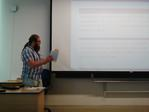42. October 9, 2018 Viktor Lopatkin (Czech Technical University in Prague) Combinatorial calculations of homology Abstract   Slides43. October 17, 2018 Andrii Khrabustovskyi (Graz University of Technology) A first look at homogenization theory Abstract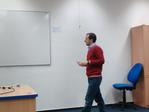44. October 23, 2018 Vsevolod Gubarev (University of Vienna) Rota-Baxter operators Abstract: We study the properties of Rota-Baxter operators (which in some sense generalize the operator of integration). We discuss the connection between Rota-Baxter operators and Yang-Baxter equation (from mathematical physics). We consider the relationships between algebras with Rota-Baxter operators and so called Loday algebras. The problem of decomposing of an algebra into a sum of two its subalgebras is involved. Slides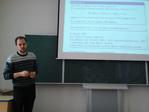45. November 13, 2018 Anna Lytova (University of Opole, Poland) On eigenvalues and singular values of adjacency matrices of regular directed random graphs Abstract   Slides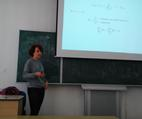46. November 27, 2018 Igor Leite Freire (Federal University of ABC, Brazil) A look on some results about Camassa-Holm type equations Abstract: In this talk we discuss some results on families of equations including the Camassa-Holm and Novikov equations. In particular, we show a 1-parameter family of equations unifying both mentioned equations. Slides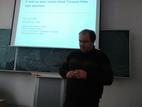47.-51. December 3-7, 2018 Andrii Khrabustovskyi (Graz University of Technology) Introduction to homogenization theory. A minicourse of 5 lectures Abstract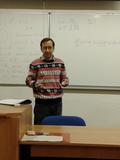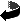Ostrava Mathematical Seminar
Created: Wed Feb 14 2018